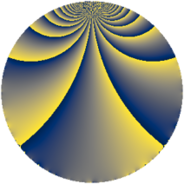# Properties

 Label 483.2.iLevel $483$ Weight $2$ Character orbit 483.i Rep. character $\chi_{483}(277,\cdot)$ Character field $\Q(\zeta_{3})$ Dimension $60$ Newform subspaces $8$ Sturm bound $128$ Trace bound $7$

# Learn more about

## Defining parameters

 Level: $$N$$ $$=$$ $$483 = 3 \cdot 7 \cdot 23$$ Weight: $$k$$ $$=$$ $$2$$ Character orbit: $$[\chi]$$ $$=$$ 483.i (of order $$3$$ and degree $$2$$) Character conductor: $$\operatorname{cond}(\chi)$$ $$=$$ $$7$$ Character field: $$\Q(\zeta_{3})$$ Newform subspaces: $$8$$ Sturm bound: $$128$$ Trace bound: $$7$$ Distinguishing $$T_p$$: $$2$$, $$5$$, $$11$$

## Dimensions

The following table gives the dimensions of various subspaces of $$M_{2}(483, [\chi])$$.

Total New Old
Modular forms 136 60 76
Cusp forms 120 60 60
Eisenstein series 16 0 16

## Trace form

 $$60q + 2q^{3} - 32q^{4} - 8q^{6} + 6q^{7} + 24q^{8} - 30q^{9} + O(q^{10})$$ $$60q + 2q^{3} - 32q^{4} - 8q^{6} + 6q^{7} + 24q^{8} - 30q^{9} - 16q^{10} + 4q^{12} - 4q^{13} - 12q^{14} + 8q^{15} - 52q^{16} - 8q^{17} - 6q^{19} - 16q^{20} - 8q^{21} + 48q^{22} - 46q^{25} + 32q^{26} - 4q^{27} + 28q^{28} + 24q^{29} + 22q^{31} - 24q^{32} - 12q^{33} - 16q^{34} + 4q^{35} + 64q^{36} - 10q^{37} - 20q^{38} + 2q^{39} - 36q^{40} + 8q^{41} + 16q^{42} - 12q^{43} - 16q^{44} - 8q^{47} - 16q^{48} + 50q^{49} - 40q^{50} + 4q^{54} - 48q^{55} - 8q^{56} + 12q^{57} + 24q^{58} - 20q^{59} + 12q^{60} - 16q^{61} + 24q^{62} - 6q^{63} + 200q^{64} + 36q^{65} - 24q^{66} - 10q^{67} - 12q^{68} - 16q^{69} + 16q^{70} + 8q^{71} - 12q^{72} + 18q^{73} - 12q^{74} + 14q^{75} + 24q^{76} + 8q^{77} - 8q^{78} - 10q^{79} + 92q^{80} - 30q^{81} + 24q^{82} + 56q^{83} + 4q^{84} - 72q^{85} - 44q^{86} + 20q^{87} - 80q^{88} + 32q^{90} - 46q^{91} - 6q^{93} - 20q^{94} - 24q^{95} + 4q^{96} + 40q^{97} - 40q^{98} + O(q^{100})$$

## Decomposition of $$S_{2}^{\mathrm{new}}(483, [\chi])$$ into newform subspaces

Label Dim. $$A$$ Field CM Traces $q$-expansion
$$a_2$$ $$a_3$$ $$a_5$$ $$a_7$$
483.2.i.a $$2$$ $$3.857$$ $$\Q(\sqrt{-3})$$ None $$0$$ $$-1$$ $$0$$ $$1$$ $$q+(-1+\zeta_{6})q^{3}+(2-2\zeta_{6})q^{4}+(2-3\zeta_{6})q^{7}+\cdots$$
483.2.i.b $$2$$ $$3.857$$ $$\Q(\sqrt{-3})$$ None $$0$$ $$-1$$ $$0$$ $$5$$ $$q+(-1+\zeta_{6})q^{3}+(2-2\zeta_{6})q^{4}+(2+\zeta_{6})q^{7}+\cdots$$
483.2.i.c $$2$$ $$3.857$$ $$\Q(\sqrt{-3})$$ None $$1$$ $$-1$$ $$-1$$ $$-5$$ $$q+\zeta_{6}q^{2}+(-1+\zeta_{6})q^{3}+(1-\zeta_{6})q^{4}+\cdots$$
483.2.i.d $$2$$ $$3.857$$ $$\Q(\sqrt{-3})$$ None $$1$$ $$-1$$ $$3$$ $$-1$$ $$q+\zeta_{6}q^{2}+(-1+\zeta_{6})q^{3}+(1-\zeta_{6})q^{4}+\cdots$$
483.2.i.e $$4$$ $$3.857$$ $$\Q(\sqrt{-3}, \sqrt{17})$$ None $$1$$ $$-2$$ $$1$$ $$10$$ $$q+\beta _{1}q^{2}+(-1+\beta _{2})q^{3}+(-2+\beta _{1}+\cdots)q^{4}+\cdots$$
483.2.i.f $$12$$ $$3.857$$ $$\mathbb{Q}[x]/(x^{12} - \cdots)$$ None $$1$$ $$6$$ $$-3$$ $$-2$$ $$q+(-\beta _{1}-\beta _{5})q^{2}+\beta _{7}q^{3}+(1+\beta _{4}+\cdots)q^{4}+\cdots$$
483.2.i.g $$16$$ $$3.857$$ $$\mathbb{Q}[x]/(x^{16} - \cdots)$$ None $$-1$$ $$-8$$ $$-5$$ $$-2$$ $$q+(\beta _{1}-\beta _{5})q^{2}+(-1-\beta _{4})q^{3}+(-2+\cdots)q^{4}+\cdots$$
483.2.i.h $$20$$ $$3.857$$ $$\mathbb{Q}[x]/(x^{20} - \cdots)$$ None $$-3$$ $$10$$ $$5$$ $$0$$ $$q+(\beta _{1}+\beta _{4})q^{2}-\beta _{9}q^{3}+(-\beta _{1}-\beta _{8}+\cdots)q^{4}+\cdots$$

## Decomposition of $$S_{2}^{\mathrm{old}}(483, [\chi])$$ into lower level spaces

$$S_{2}^{\mathrm{old}}(483, [\chi]) \cong$$ $$S_{2}^{\mathrm{new}}(21, [\chi])$$$$^{\oplus 2}$$$$\oplus$$$$S_{2}^{\mathrm{new}}(161, [\chi])$$$$^{\oplus 2}$$